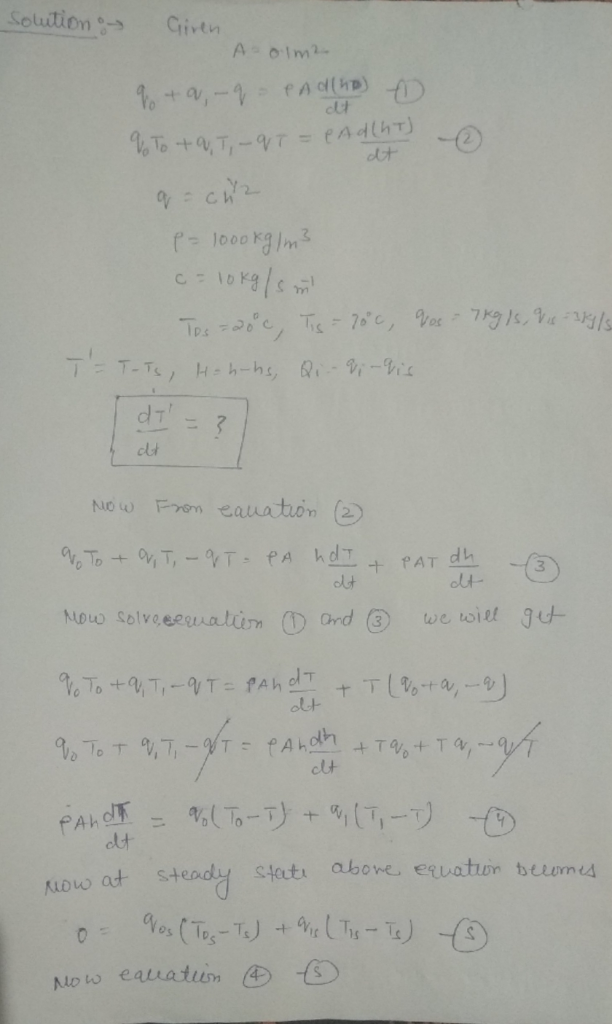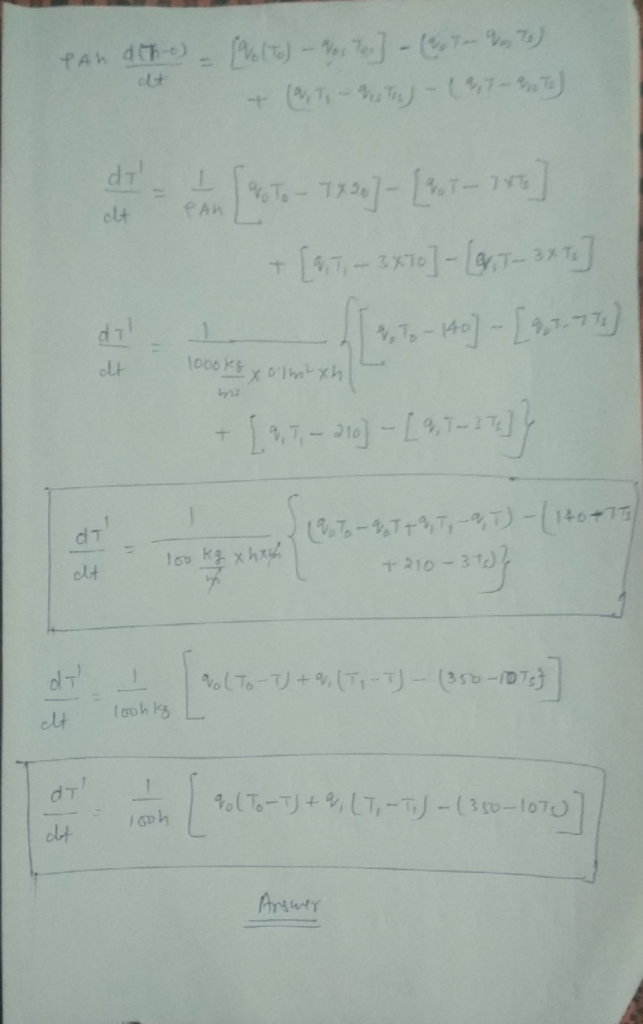Homework Help Question & Answers

The area of the stirred tank heater is 0.1m^2 and the dynamic model of it are q0+q1-q=ρA*d(h)/d(t...

The area of the stirred tank heater is 0.1m^2 and the dynamic model of it are
q0+q1-q=ρA*d(h)/d(t)
q0T0+q1T1-qT= ρA*d(hT)/d(t)

and the values are q=c*h^(1/2), ρ=1000kg/m^3, c=10kg/s*m^(-1), T0s= 20°C, T1s= 70°C, q0s= 7kg/s, q1s= 3kg/s

If T'=T-Ts, H=h-hs, Qi= qi-qis, and so on, find dT'/dt for the step change of q1.Add Answer of: The area of the stirred tank heater is 0.1m^2 and the dynamic model of it are q0+q1-q=ρA*d(h)/d(t...
More Homework Help Questions Additional questions in this topic.

• Consider the following estimated models: Model 1: y-16 + 5.42x Model 2: y-29 + 29 In(x) Model 3: In(y) 2.0+0.10x, se 0.06 Model 4: In(y -2.4+0.36 In(; se 0.12 b. For each model, what is the predicted...

Need Online Homework Help?

Get FREE EXPERT Answers
WITHIN MINUTES
Related Questions